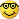# Better NN EA development - page 4422

Can you please explain to me in short what is this software? and how efficient is the random number generator within it?

LittleWalker.86

Hi,

I really didn't test these programs,

I saw them in FF , and attached them here , might be useful,

and if you are a coder,then you can help Mr Barnix for coding good strategy tester for his Better EA,

I am coding now a trade training algorithm, but it need massive effort and time to completion,

thx.1310

SVM_EA_v25c_

Forwardtest demo

Files:1310

Should I normalize/standardize/rescale the

First, some definitions. "Rescaling" a vector means to add or subtract a

constant and then multiply or divide by a constant, as you would do to

change the units of measurement of the data, for example, to convert a

temperature from Celsius to Fahrenheit.

"Normalizing" a vector most often means dividing by a norm of the vector,

for example, to make the Euclidean length of the vector equal to one. In the

NN literature, "normalizing" also often refers to rescaling by the minimum

and range of the vector, to make all the elements lie between 0 and 1.

"Standardizing" a vector most often means subtracting a measure of location

and dividing by a measure of scale. For example, if the vector contains

random values with a Gaussian distribution, you might subtract the mean and

divide by the standard deviation, thereby obtaining a "standard normal"

random variable with mean 0 and standard deviation 1.

Two of the most useful ways to standardize inputs are:

o Mean 0 and standard deviation 1

o Midrange 0 and range 2 (i.e., minimum -1 and maximum 1)

Note that statistics such as the mean and standard deviation are computed

from the training data, not from the validation or test data. The validation

and test data must be standardized using the statistics computed from the

training data.

Formulas are as follows:

Files:
io_scaling.pdf  34 kb
io_1.jpg  18 kb
io_2.jpg  13 kb308

barnix:
Should I normalize/standardize/rescale the

First, some definitions. "Rescaling" a vector means to add or subtract a

constant and then multiply or divide by a constant, as you would do to

change the units of measurement of the data, for example, to convert a

temperature from Celsius to Fahrenheit.

"Normalizing" a vector most often means dividing by a norm of the vector,

for example, to make the Euclidean length of the vector equal to one. In the

NN literature, "normalizing" also often refers to rescaling by the minimum

and range of the vector, to make all the elements lie between 0 and 1.

"Standardizing" a vector most often means subtracting a measure of location

and dividing by a measure of scale. For example, if the vector contains

random values with a Gaussian distribution, you might subtract the mean and

divide by the standard deviation, thereby obtaining a "standard normal"

random variable with mean 0 and standard deviation 1.

Two of the most useful ways to standardize inputs are:

o Mean 0 and standard deviation 1

o Midrange 0 and range 2 (i.e., minimum -1 and maximum 1)

Note that statistics such as the mean and standard deviation are computed

from the training data, not from the validation or test data. The validation

and test data must be standardized using the statistics computed from the

training data.

Formulas are as follows:

THANKS barnix, i love ur work... its keep me inspired...hmm barnix do you've read this site

explanation from Paco Hernandez Gomez... Similar with your PNN training ....

regards,

MANSTIR1310

Files:221310

1-Tester_v04_5_EURUSD

2-SVM_EA_v25c

Files:1310

1-Tester_v04_5a_EURUSD

standardize with range

2-Tester_v04_5_EURUSD

standardize with med,std

Files:1310

1-Tester_v04_5b_EURUSD

standardize with range calculated from train data

2-Tester_v04_5_EURUSD

standardize with med,std

Files: